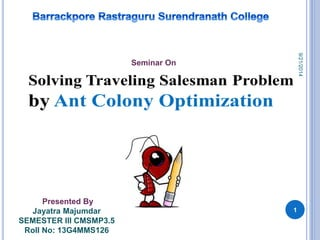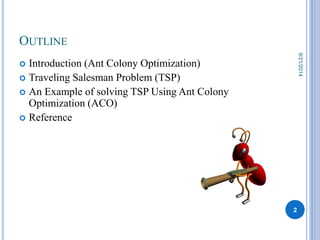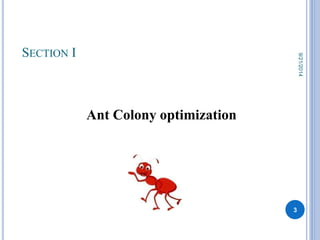Successfully reported this slideshow.

# Show ant-colony-optimization-for-solving-the-traveling-salesman-problem

19

Share×
1 of 35
1 of 35

# Show ant-colony-optimization-for-solving-the-traveling-salesman-problem

19

Share

A example of Travelling salesman problem solved using ANT COLONY OPTIMIZATION.

A example of Travelling salesman problem solved using ANT COLONY OPTIMIZATION.

## More Related Content

### Related Books

Free with a 14 day trial from Scribd

See all

### Related Audiobooks

Free with a 14 day trial from Scribd

See all

### Show ant-colony-optimization-for-solving-the-traveling-salesman-problem

1. 1. Presented By Jayatra Majumdar SEMESTER III CMSMP3.5 Roll No: 13G4MMS126 Seminar On 1 9/21/2014
2. 2. OUTLINE  Introduction (Ant Colony Optimization)  Traveling Salesman Problem (TSP)  An Example of solving TSP Using Ant Colony Optimization (ACO)  Reference 2 9/21/2014
3. 3. SECTION I Ant Colony optimization 3 9/21/2014
4. 4. WHAT IS ANT COLONY OPTIMIZATION (ACO) ? Ant Colony Optimization (ACO) studies artificial systems that take inspiration from the behavior of real ant colonies and which are used to solve discrete optimization problems. In 1991, the Ant Colony Optimization metaheuristic was defined by Dorigo, Di Caro and Gambardella. 4 9/21/2014
5. 5. SECTION II Travelling Salesman Problem (TSP) 5 9/21/2014
6. 6. TRAVELING SALESMAN PROBLEM (TSP) Informally: The problem of finding the shortest tour through a set of cities starting at some city and going through all other cities once and only once, returning to the starting city Formally: Finding the shortest Hamiltonian path in a fully-connected graph TSP belongs to the class of NP-complete problems. Thus, it is assumed that there is no efficient algorithm for solving TSPs. 6 9/21/2014
7. 7. A Hamiltonian path (or traceable path) is a path in an undirected graph which visits each vertex exactly once. A Hamiltonian cycle (or Hamiltonian circuit) is a cycle in an undirected graph which visits each vertex exactly once and also returns to the starting vertex. Determining whether such paths and cycles exist in graphs is the Hamiltonian path problem which is NP-complete. A graph representing the vertices, edges, and faces of a dodecahedron, with a Hamiltonian cycle shown by red color edges. NP problem can not be solved in polynomial time. 7 9/21/2014
8. 8. TECHNIQUES FOR SOLVING TSP TSP can be solved through various way. Some of those are,  Exact algorithms: The most direct solution would be to try all permutations (ordered combinations) and see which one is cheapest (using brute force search).  Computational complexity  Complexity of approximation  Heuristic and approximation algorithms  4.3.1 Constructive heuristics  4.3.2 Iterative improvement  4.3.3 Randomized improvement • 4.3.3.1 Ant colony optimization  Special cases  4.4.1 Metric TSP  4.4.2 Euclidean TSP  4.4.3 Asymmetric TSP  4.4.3.1 Solving by conversion to symmetric TSP, etc. 8 9/21/2014
9. 9. SECTION III An Example of solving TSP Using Ant Colony Optimization (ACO) 9 9/21/2014
10. 10. A simple TSP example [] 1 [] [] 2 3 [] 4 [] 5 Distances Between Cities: dAB =100; dAD = 50; dAE = 70; dAC = 60; dBE = 60; dBC = 60; dCD = 90; dCE = 40; dDE = 150; A E D C B Here, m=n Where, m= number of ants; n= number of cities 9/21/2014 10
11. 11. ITERATION 1 A E D C B [A] 1 [B] [E] 5 [C] 3 2 [D] 4 And at first iteration , each of them are assigned to different city. 9/21/2014 11
12. 12. HOW TO BUILD NEXT SUB-SOLUTION? [A] 1 A D B E C A1 has four different option i.e. AB=100,AC=60, AD=50 and AE=70 A1 will choose path by a probabilistic value   ( t )   .          k  ij ij ij k Ji t    ( ) .  il il p t  ( ) Depending on a random number. 12 9/21/2014
13. 13. [A] τk = ij Amount of Pheromone ηij k= Reciprocal of the distance The amount of pheromone on edge(A,B),edge(A,C), edge(A,D) and edge(A,E) are τA,B(t), τA,C(t), τA,D(t) and τA,E(t) respectively with same initial value 0.2. The value of η(A,B), η(A,C), η(A,D) and η(A,E) are 1/100, 1/60, 1/50 and 1/70 respectively. Value of α=0.2 and β=0.6 Now the probabilities , , ,and are as shown here respectively. HOW TO BUILD NEXT SUB-SOLUTION? A D B E C Depending on a random number A1 choose AD as path. Assuming random no.=0.25 1 k (t) AB p k (t) AE (t) p k AD (t) p k AC p                     (t) 0.3056 k AE p 0.6 . 1/70 0.2 0.2 0.6 . 1/50 0.2 0.2 0.6 . 1/60 0.2 0.2 0.6 . 1/100 0.2 0.2 0.6 * 1/70 0.2 0.2 (t) k AE p          0.1791 AB 0.6 * 1/100 0.2 0.2 AB                     (t) 0.2432 k AC p 0.6 . 1/70 0.2 0.2 0.6 . 1/50 0.2 0.2 0.6 . 1/60 0.2 0.2 0.6 . 1/100 0.2 0.2 0.6 * 1/60 0.2 0.2 (t) k AC p      0.2719 AD 50 AD 13 9/21/2014
14. 14. EVAPORATION RATE IN ITERATION 1 14 m  ij ij ij t t t Pheromone decay:  ( )  (1   ). ( )    ( ) k 1 Iteration Path Decay Rate 1 AB 0.08 9/21/2014 AC 0.08 AD 0.08 AE 0.08 BC 0.08 BD 0.08 BE 0.08 CD 0.08 CE 0.08 DE 0.08 Assume that ρ =0.6
15. 15. ITERATION 2 [A] 1 [A] 1 [A] 1 [A,D] 1 A D B E C 15 9/21/2014
16. 16. HOW TO BUILD NEXT SUB-SOLUTION? A [A,D] D B E C Now A1 has three different option i.e. DB=70,DC=90,DE=150 Again, A1 will choose path by the probabilistic value   ( t )   .          k  ij ij ij k Ji t    ( ) .  il il p t  ( ) Depending on a random number. 1 16 9/21/2014
17. 17. The amount of pheromone on edge(D,B) ,edge(D,C) and edge(D,E) are τD,B(t),τD,C(t) and τD,E(t) respectively with initial value 0.2. The pheromone on path AD after first iteration will be updated by. HOW TO BUILD NEXT SUB-SOLUTION? A [A,D] D B E C    / ( ) ( , ) ( ) 0. 100/ 50 2  SO , A   AD A    AD Assuming a random number = 0.32, A1 choose DC as path. t else k t if i j T k Q L k ij  Where, Q=100 and LK =50 1 Value of α=0.2 and β=0.6 ( ) A p t DC And the probabilities , and p A ( t ) DE are as shown here respectively. ( ) A p t DB 0.4009 DE DB 70 DB                 (t) 0.3461 k DC p 0.6 . 1/70 0.2 0.2 0.6 . 1/150 0.2 0.2 0.6 . 1/90 0.2 0.2 0.6 * 1/90 0.2 0.2 (t) k DC p             (t) 0.2528 . 1/70 0.2 0.2 0.6 . 1/50 0.2 0.2 0.6 150 (t) DE    17 9/21/2014
18. 18. EVAPORATION RATE IN ITERATION 2 18 m  ij ij ij t t t Pheromone decay:  ( )  (1   ). ( )    ( ) k 1 Iteration Path Decay Rate 2 AB 0.032 9/21/2014 AC 0.032 AD 0.832 AE 0.032 BC 0.032 BD 0.032 BE 0.032 CD 0.032 CE 0.032 DE 0.032 Assume that ρ =0.6
19. 19. ITERATION 3 A D B E [A,D,C] C [A,D] 1 [A,D] 1 1 19 9/21/2014
20. 20. HOW TO BUILD NEXT SUB-SOLUTION? A D B E [A,D,C] C Now A1 has another two option i.e. CE=40,CB=60 Again, A1 will choose path by the probabilistic value   ( t )   .          k  ij ij ij k Ji t    ( ) .  il il p t  ( ) Depending on a random number. 1 20 9/21/2014
21. 21. [A,D,C] 1 The amount of pheromone on Edge(C,B) and edge(C,E) are τC,B(t) and τC,E(t) respectively with initial value 0.2. The pheromone on path DC after second iteration will be updated by. HOW TO BUILD NEXT SUB-SOLUTION? A D B E C    / ( ) ( , ) ( ) 0. 100/ 140 0.7142  SO , A    DC A   DC ( ) A p t CB Assuming a random number =0.5, A1 choose CE as path. t else k t if i j T k Q L k ij  Where, Q=100 and LK =50+90 =140 Value of α=0.2 and β=0.6 And the probabilities and p A ( t ) CE are as shown here respectively.             (t) 0.4386 CB k p 0.6 . 1/40 0.2 0.2 0.6 . 1/60 0.2 0.2 0.6 * 1/60 0.2 0.2 (t) k p CB                0.5613 0.6 . 1/40 0.2 0.2 0.6 . 1/60 0.2 0.2 0.6 * 1/40 0.2 0.2 (t) CE CE   21 9/21/2014
22. 22. EVAPORATION RATE IN ITERATION 3 22 m  ij ij ij t t t Pheromone decay:  ( )  (1   ). ( )    ( ) k 1 Iteration Path Decay Rate 3 AB 0.0128 9/21/2014 AC 0.0128 AD 0.3328 AE 0.0128 BC 0.0128 BD 0.0128 BE 0.0128 CD 0.2984 CE 0.0128 DE 0.0128 Assume that ρ =0.6
23. 23. ITERATION 4 A D B E [A,D,C] C 1 [A,D,C] 1 [A,D,C,E] 1 23 9/21/2014
24. 24. HOW TO BUILD NEXT SUB-SOLUTION? A D B E C Now A1 has only one path i.e. EB=60 as A1 has already visited city A,C and D. Again, A1 will choose path by the probabilistic value   ( t )   .          k  ij ij ij k Ji t    ( ) .  il il p t  ( ) Depending on a random number. [A,D,C,E] 1 24 9/21/2014
25. 25. The amount of pheromone on Edge(E,B) is τE,B(t) with initial value 0.2. The pheromone on path CE after fourth iteration will be updated by. HOW TO BUILD NEXT SUB-SOLUTION? A D B E C    / ( ) ( , ) ( ) 0. 100/ 180 0.5555  SO , A    CE A   CE ( ) A p t EB Assuming a random number =0.5, A1 choose EB as path. t else k t if i j T k Q L k ij  Where, Q=100 and LK =50+90+40 =180 Value of α=0.2 and β=0.6 And the probabilities is as shown here, [A,D,C,E] 1          (t) 1 k p 0.6 . 1/60 0.2 0.2 0.6 * 1/60 0.2 0.2 (t) k EB p EB  25 9/21/2014
26. 26. EVAPORATION RATE IN ITERATION 4 26 m  ij ij ij t t t Pheromone decay:  ( )  (1   ). ( )    ( ) k 1 Iteration Path Decay Rate 4 AB 0.00512 9/21/2014 AC 0.00512 AD 0.13312 AE 0.00512 BC 0.00512 BD 0.00512 BE 0.00512 CD 0.11936 CE 0.22732 DE 0.00512 Assume that ρ =0.6
27. 27. ITERATION 5 A D B E C [A,D,C,E,B] [A,D,C,E] 1 [A,D,C,E] 1 1 27 9/21/2014
28. 28. HOW TO BUILD NEXT SUB-SOLUTION? A D [A,D,C,E,B] B E C A1 now has only one path i.e. BA=100 as A1 has already visited all other city and return to home city. Again, A1 will choose path by the probabilistic value   ( t )   .          k  ij ij ij k Ji t    ( ) .  il il p t  ( ) Depending on a random number. 1 28 9/21/2014
29. 29. The amount of pheromone on Edge(B,A) is τBA(t) with initial value 0.2. The pheromone on path EB after fifth iteration will be updated by. HOW TO BUILD NEXT SUB-SOLUTION? A D [A,D,C,E,B] B E C    / ( ) ( , ) ( ) 0. 100/ 240 0.4166  SO , A    EB A   E ( ) A p t BA Assuming a random number=0.8, A1 choose BA as path. t else k t if i j T k Q L k ij  B Where, Q=100 and LK= 50+90+40+60 =240 Value of α=0.2 and β=0.6 And the probabilities is as shown here, 1          (t) 1 k p 0.6 . 1/100 0.2 0.2 0.6 * 1/100 0.2 0.2 (t) k BA p BA  29 9/21/2014
30. 30. EVAPORATION RATE IN ITERATION 5 30 m  ij ij ij t t t Pheromone decay:  ( )  (1   ). ( )    ( ) k 1 Iteration Path Decay Rate 4 AB 0.002048 9/21/2014 AC 0.002048 AD 0.053248 AE 0.002048 BC 0.002048 BD 0.002048 BE 0.16868 CD 0.047744 CE 0.090928 DE 0.002048 Assume that ρ =0.6
31. 31. ITERATION 6 [A,D,C,E,B,A] A D B E [A,D,C,E,B] C [A,D,C,E,B] 1 [A,D,C,E,B] 1 1 1 Indicated path is the optimal path for ant A1 . 31 9/21/2014
32. 32. PATH FOR EACH ANT AND PATH LENGTH [A,D,C,E,B,A] 1 L1=50+90+40+60+100 L1 =340 L2 =60+90+50+70+60 L2 =330 L3 =60+60+150+50+60 L3 =380 L4 =150+70+100+60+90 L4 =470 L5 =70+100+60+90+150 L5 = 470 [B,C,D,A,E.B] 2 [C,B,E,D,A,C] 3 [D,E,A,B,C,D] 4 [E,A,B,C,D,E] 5 Similarly, path for other ants are presented here with optimal path length. 9/21/2014 32
33. 33. REFERENCES  E. Bonabeau, M. Dorigo, G. Theraulaz. Swarm Intelligence: From Natural to Artificial Systems, 1999  M. Dorigo and L. Gambardella. Ant colony system: A cooperative learning approach to the traveling salesman problem, 1997.  M. Dorigo and T. Stützle. Ant Colony Optimization, MIT Press, 2004.  J. Kennedy and R. Eberhart. Swarm Intelligence, 2001 9/21/2014 33
34. 34. Thank you for yours attention 9/21/2014 34
35. 35. Any Question? 9/21/2014 35